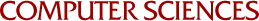Minimum Principle Sufficiency

Michael C Ferris and Olvi L Mangasarian
1989

We characterize the property of obtaining a solution to a convex program by minimizing over the feasible region a linearization of the objective function at any of its solution points (Minimum Principle Sufficiency). For the case of a monotone linear complementarity problem this MPS property is completely equivalent to the existence of a nondegenerate solution to the problem. For the case of a convex quadratic program the MPS property is equivalent to the span of the Hessian of the objective function being contained in the normal cone to the feasible region at any solution point plus the cone generated by the gradient of the objective function at any solution point. This in turn is equivalent to the quadratic program having a weak sharp minimum. An important application of the MPS property is that minimizing on the feasible region a linearization of the objective function at a point in a neighborhood of a solution point gives an exact solution of the convex program. This leads to finite termination of convergent algorithms that periodically minimize such a linearization.